The Perceptron

Video II

Assumptions

1. Binary classification (i.e. $y_i \in \{-1, +1\}$)
2. Data is linearly separable

Classifier

$$h(x_i) = \textrm{sign}(\mathbf{w}^\top \mathbf{x}_i + b)$$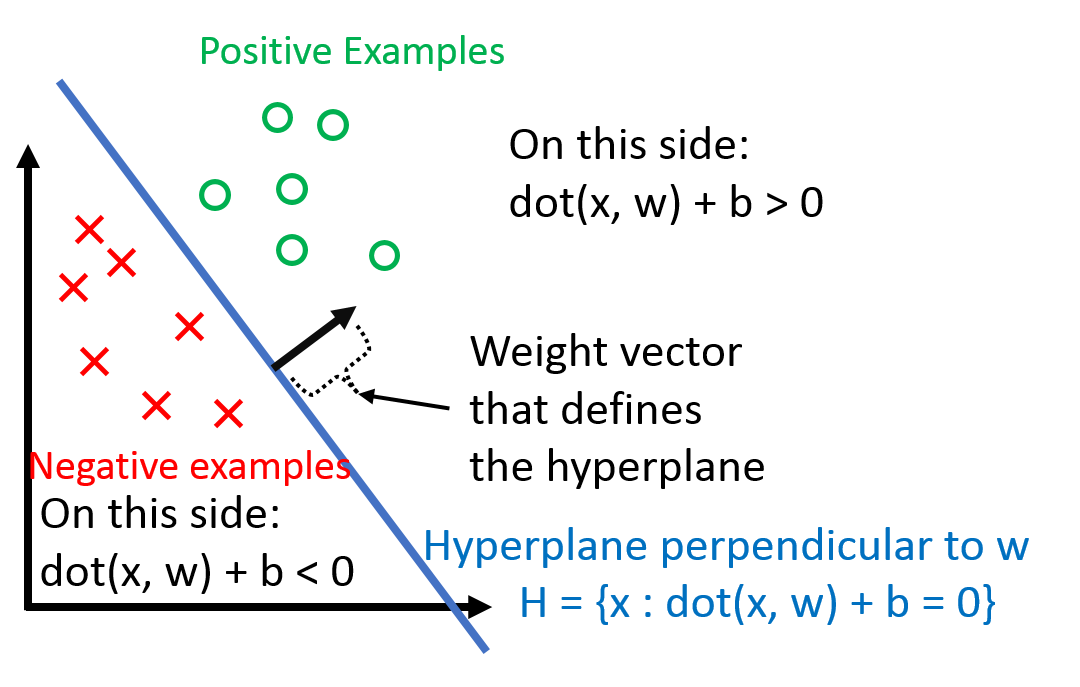$b$ is the bias term (without the bias term, the hyperplane that $\mathbf{w}$ defines would always have to go through the origin). Dealing with $b$ can be a pain, so we 'absorb' it into the feature vector $\mathbf{w}$ by adding one additional constant dimension. Under this convention, $$\mathbf{x}_i \hspace{0.1in} \text{becomes} \hspace{0.1in} \begin{bmatrix} \mathbf{x}_i \\ 1 \end{bmatrix} \\ \mathbf{w} \hspace{0.1in} \text{becomes} \hspace{0.1in} \begin{bmatrix} \mathbf{w} \\ b \end{bmatrix} \\$$ We can verify that $$\begin{bmatrix} \mathbf{x}_i \\ 1 \end{bmatrix}^\top \begin{bmatrix} \mathbf{w} \\ b \end{bmatrix} = \mathbf{w}^\top \mathbf{x}_i + b$$ Using this, we can simplify the above formulation of $h(\mathbf{x}_i)$ to $$h(\mathbf{x}_i) = \textrm{sign}(\mathbf{w}^\top \mathbf{x})$$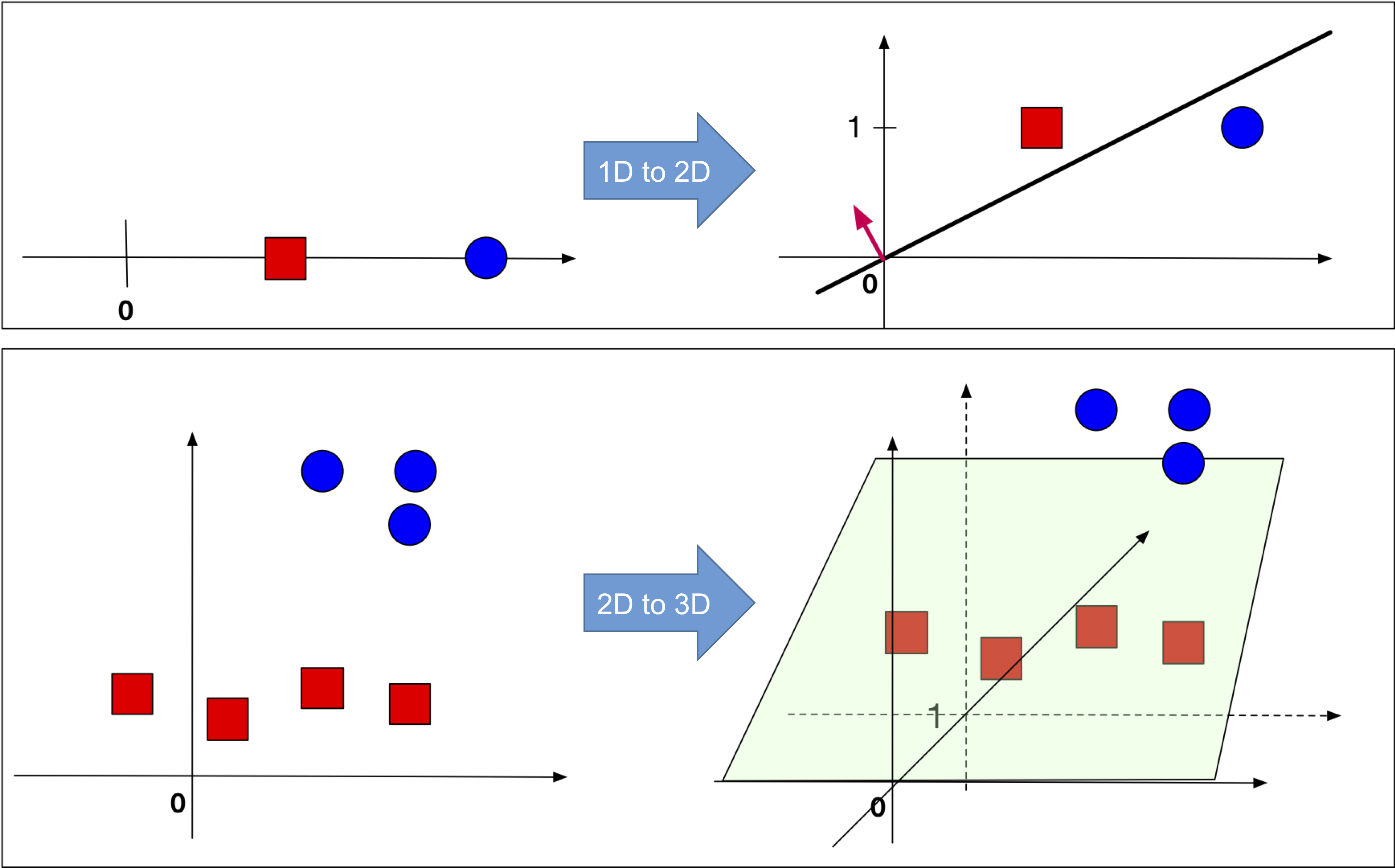(Left:) The original data is 1-dimensional (top row) or 2-dimensional (bottom row). There is no hyper-plane that passes through the origin and separates the red and blue points. (Right:) After a constant dimension was added to all data points such a hyperplane exists.
Observation: Note that $$y_i(\mathbf{w}^\top \mathbf{x}_i) > 0 \Longleftrightarrow \mathbf{x}_i \hspace{0.1in} \text{is classified correctly}$$ where 'classified correctly' means that $x_i$ is on the correct side of the hyperplane defined by $\mathbf{w}$. Also, note that the left side depends on $y_i \in \{-1, +1\}$ (it wouldn't work if, for example $y_i \in \{0, +1\}$).

Perceptron Algorithm

Now that we know what the $\mathbf{w}$ is supposed to do (defining a hyperplane the separates the data), let's look at how we can get such $\mathbf{w}$.

Perceptron Algorithm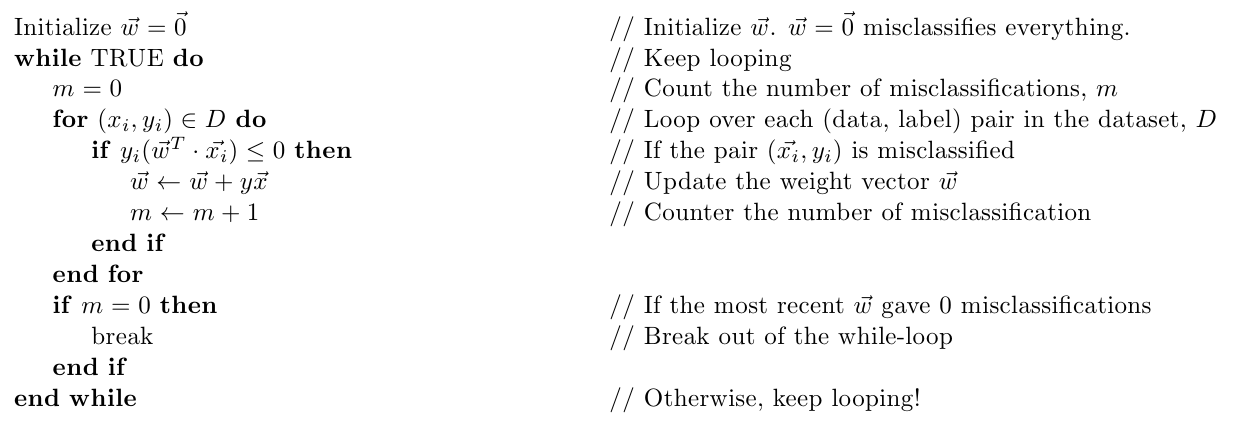Geometric Intuition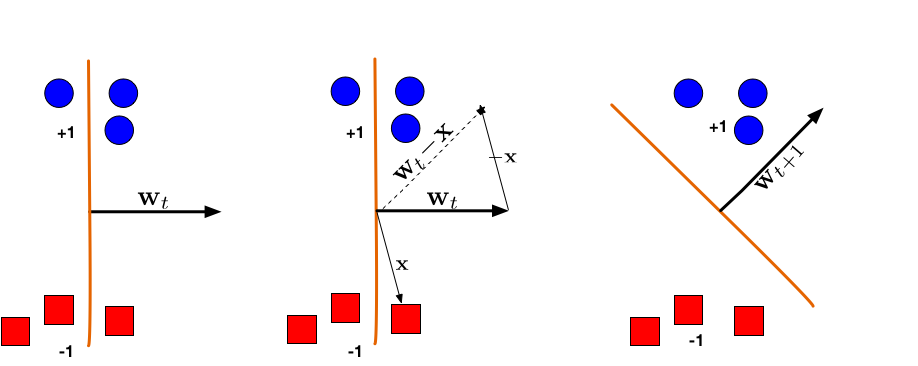Illustration of a Perceptron update. (Left:) The hyperplane defined by $\mathbf{w}_t$ misclassifies one red (-1) and one blue (+1) point. (Middle:) The red point $\mathbf{x}$ is chosen and used for an update. Because its label is -1 we need to subtract $\mathbf{x}$ from $\mathbf{w}_t$. (Right:) The udpated hyperplane $\mathbf{w}_{t+1}=\mathbf{w}_t-\mathbf{x}$ separates the two classes and the Perceptron algorithm has converged.

Quiz: Assume a data set consists only of a single data point $\{(\mathbf{x},+1)\}$. How often can a Perceptron misclassify this point $\mathbf{x}$ repeatedly? What if the initial weight vector $\mathbf{w}$ was initialized randomly and not as the all-zero vector?

Perceptron Convergence

The Perceptron was arguably the first algorithm with a strong formal guarantee. If a data set is linearly separable, the Perceptron will find a separating hyperplane in a finite number of updates. (If the data is not linearly separable, it will loop forever.)

The argument goes as follows: Suppose $\exists \mathbf{w}^*$ such that $y_i(\mathbf{x}^\top \mathbf{w}^* ) > 0$ $\forall (\mathbf{x}_i, y_i) \in D$.

Now, suppose that we rescale each data point and the $\mathbf{w}^*$ such that $$||\mathbf{w}^*|| = 1 \hspace{0.3in} \text{and} \hspace{0.3in} ||\mathbf{x}_i|| \le 1 \hspace{0.1in} \forall \mathbf{x}_i \in D$$ Let us define the Margin $\gamma$ of the hyperplane $\mathbf{w}^*$ as $\gamma = \min_{(\mathbf{x}_i, y_i) \in D}|\mathbf{x}_i^\top \mathbf{w}^* |$.

A little observation (which will come in very handy): For all $\mathbf{x}$ we must have $y(\mathbf{x}^\top \mathbf{w}^*)=|\mathbf{x}^\top \mathbf{w}^*|\geq \gamma$. Why? Because $\mathbf{w}^*$ is a perfect classifier, so all training data points $(\mathbf{x},y)$ lie on the "correct" side of the hyper-plane and therefore $y=sign(\mathbf{x}^\top \mathbf{w}^*)$. The second inequality follows directly from the definition of the margin $\gamma$.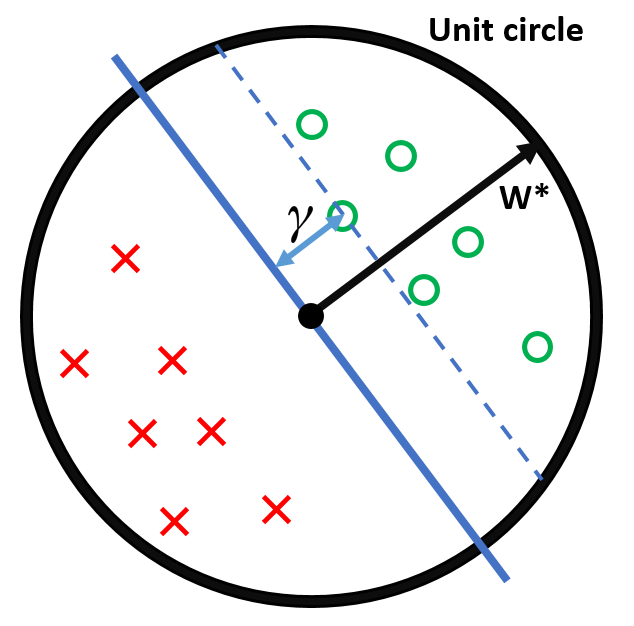To summarize our setup:
• All inputs $\mathbf{x}_i$ live within the unit sphere
• There exists a separating hyperplane defined by $\mathbf{w}^*$, with $\|\mathbf{w}\|^*=1$ (i.e. $\mathbf{w}^*$ lies exactly on the unit sphere).
• $\gamma$ is the distance from this hyperplane (blue) to the closest data point.

Theorem: If all of the above holds, then the Perceptron algorithm makes at most $1 / \gamma^2$ mistakes.

Proof:
Keeping what we defined above, consider the effect of an update ($\mathbf{w}$ becomes $\mathbf{w}+y\mathbf{x}$) on the two terms $\mathbf{w}^\top \mathbf{w}^*$ and $\mathbf{w}^\top \mathbf{w}$. We will use two facts:
• $y( \mathbf{x}^\top \mathbf{w})\leq 0$: This holds because $\mathbf x$ is misclassified by $\mathbf{w}$ - otherwise we wouldn't make the update.
• $y( \mathbf{x}^\top \mathbf{w}^*)>0$: This holds because $\mathbf{w}^*$ is a separating hyper-plane and classifies all points correctly.
1. Consider the effect of an update on $\mathbf{w}^\top \mathbf{w}^*$: $$(\mathbf{w} + y\mathbf{x})^\top \mathbf{w}^* = \mathbf{w}^\top \mathbf{w}^* + y(\mathbf{x}^\top \mathbf{w}^*) \ge \mathbf{w}^\top \mathbf{w}^* + \gamma$$ The inequality follows from the fact that, for $\mathbf{w}^*$, the distance from the hyperplane defined by $\mathbf{w}^*$ to $\mathbf{x}$ must be at least $\gamma$ (i.e. $y (\mathbf{x}^\top \mathbf{w}^*)=|\mathbf{x}^\top \mathbf{w}^*|\geq \gamma$).

This means that for each update, $\mathbf{w}^\top \mathbf{w}^*$ grows by at least $\gamma$.
2. Consider the effect of an update on $\mathbf{w}^\top \mathbf{w}$: $$(\mathbf{w} + y\mathbf{x})^\top (\mathbf{w} + y\mathbf{x}) = \mathbf{w}^\top \mathbf{w} + \underbrace{2y(\mathbf{w}^\top\mathbf{x})}_{<0} + \underbrace{y^2(\mathbf{x}^\top \mathbf{x})}_{0\leq \ \ \leq 1} \le \mathbf{w}^\top \mathbf{w} + 1$$ The inequality follows from the fact that
• $2y(\mathbf{w}^\top \mathbf{x}) < 0$ as we had to make an update, meaning $\mathbf{x}$ was misclassified
• $0\leq y^2(\mathbf{x}^\top \mathbf{x}) \le 1$ as $y^2 = 1$ and all $\mathbf{x}^\top \mathbf{x}\leq 1$ (because $\|\mathbf x\|\leq 1$).
3. This means that for each update, $\mathbf{w}^\top \mathbf{w}$ grows by at most 1.
4. Now remember from the Perceptron algorithm that we initialize $\mathbf{w}=\mathbf{0}$. Hence, initially $\mathbf{w}^\top\mathbf{w}=0$ and $\mathbf{w}^\top\mathbf{w}^*=0$ and after $M$ updates the following two inequalities must hold:
• (1) $\mathbf{w}^\top\mathbf{w}^*\geq M\gamma$
• (2) $\mathbf{w}^\top \mathbf{w}\leq M$.
We can then complete the proof: \begin{align} M\gamma &\le \mathbf{w}^\top \mathbf{w}^* &&\text{By (1)} \\ &=\|\mathbf{w}\|\cos(\theta) && \text{by definition of inner-product, where $\theta$ is the angle between $\mathbf{w}$ and $\mathbf{w}^*$.}\\ &\leq ||\mathbf{w}|| &&\text{by definition of $\cos$, we must have $\cos(\theta)\leq 1$.} \\ &= \sqrt{\mathbf{w}^\top \mathbf{w}} && \text{by definition of $\|\mathbf{w}\|$} \\ &\le \sqrt{M} &&\text{By (2)} \\ & \textrm{ }\\ &\Rightarrow M\gamma \le \sqrt{M} \\ &\Rightarrow M^2\gamma^2 \le M \\ &\Rightarrow M \le \frac{1}{\gamma^2} && \text{And hence, the number of updates $M$ is bounded from above by a constant.} \end{align}

Quiz: Given the theorem above, what can you say about the margin of a classifier (what is more desirable, a large margin or a small margin?) Can you characterize data sets for which the Perceptron algorithm will converge quickly? Draw an example.

History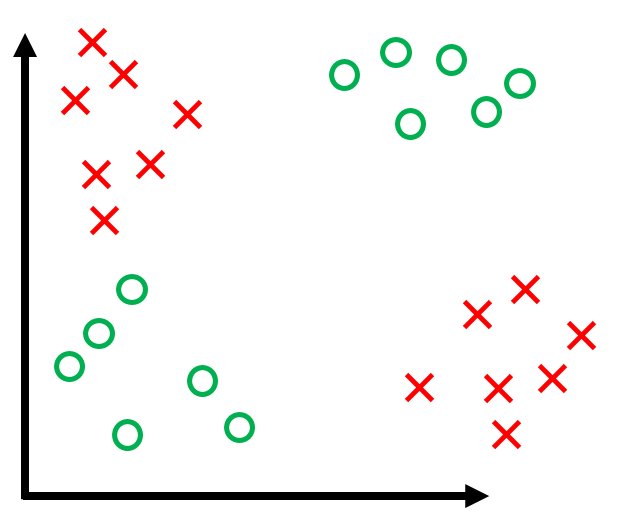• Initially, huge wave of excitement ("Digital brains") (See The New Yorker December 1958)
• Then, contributed to the A.I. Winter. Famous example of a simple non-linearly separable data set, the XOR problem (Minsky 1969):